﻿ ASP NET, NET Framework, SQL, Visual Studio | Professional Programming | Viacheslav Eremin
(MVC) MVC (2019)

## Generic VB.NET function for loading ExcelFile to DataBase (EF6 CodeFirst) with Reflection and avoiding Linq-to-Entity Linq.Expressions (use Linq-to-Object IEnumerable)

In my current project I need to load to DB a lot of Excel files with a lot of separate tables in each sheet.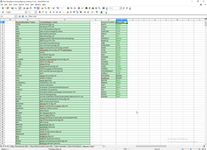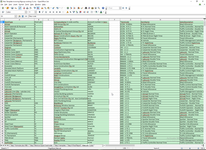I write simple loader in ASP.NET 5. This time is only start of project - I do not create a report, and I has markup of loading only first 5 tables of first XLS file.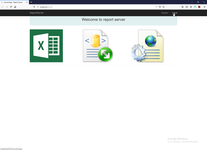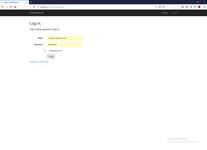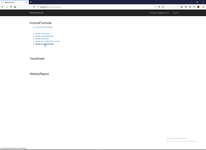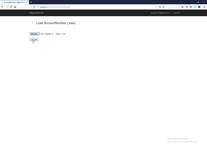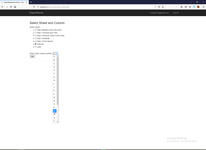This is commmon description of logic of my loader. It's so simple and I do not publish its code, for target reader of my articles is more than enough.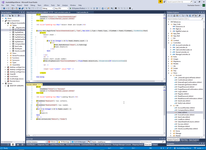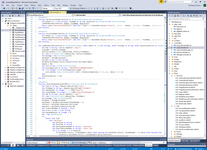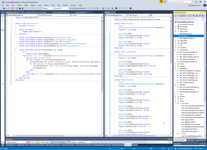Firstly I write loader to db in simplest as possible way.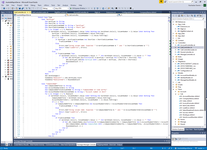After I finish written second loader I stopped for thinking. It is possible to write universal loader for dozens various tables?

And finally I has creating code below, its common and working fine in all case of my project and allow me to change raw text from Excel call to key during loading process.

`   1:  Imports OfficeOpenXml`
`   2:  Imports System.Data.Entity`
`   3:  Imports System.Linq.Expressions`
`   4:  Imports System.Reflection`
`   5:   `
`   6:  Namespace Controllers`
`   7:   `
`   8:      Public Class Xls`
`   9:          'Example of call`
`  10:          '`
`  11:          'Dim CurRowValue() As String = {"", ""}`
`  12:          'Dim ColumnNames() As String = {"Account number", "New code"}`
`  13:          'If XlsLoader.TwoColumnLoader(Of MgtlDbContext, AccountNumber)(WorkSheet, ColumnNumber, RowCount,`
`  14:          '                                                                 ColumnNames, CurRowValue,`
`  15:          '                                                                 db1, db1.AccountNumbers,`
`  16:          '                                                                 Function(X) X.AccountNumberCol = CurRowValue(0) And X.NewCode = CurRowValue(1),`
`  17:          '                                                                 Errors, RowsAddedCount) Then`
`  18:          '     ViewData("RowCount") = db1.AccountNumbers.Count`
`  19:          '     ViewData("RowCountAdd") = RowsAddedCount`
`  20:          '    Return View("Success", Errors)`
`  21:          'Else`
`  22:          '    Return View("LoadError", Errors)`
`  23:          'End If`
`  24:          '`
`  25:          'All cell must have data`
`  26:          '`
`  27:          Public Delegate Function KeyCallBack(Of T As {Class, New})(X As String(), ByRef Y As List(Of String)) As T`
`  28:   `
`  29:          Public Shared Function Loader(Of Db As DbContext, T As {Class, New})(WorkSheet As ExcelWorksheet,`
`  30:                                           StartColumnNumber As Integer,`
`  31:                                           MaxRowsCount As Integer,`
`  32:                                           ByVal ColumnNames() As String,`
`  33:                                           ByRef CurRowValue() As String,`
`  34:                                           ByVal db1 As Db, ByVal DbSet As DbSet(Of T),`
`  35:                                           ByVal FilterPredicate As Func(Of T, Boolean), ' LINQ to Object instead LINQ to Entities - ByVal FilterPredicate As Expression(Of Func(Of T, Boolean)), `
`  36:                                           ByRef Errors As List(Of String),`
`  37:                                           ByRef RowsAddedCount As Integer,`
`  38:                                           Optional AllowEmptyCell As Boolean = False,`
`  39:                                           Optional ByRef CreateKeyCallBack As KeyCallBack(Of T) = Nothing ' As Func(Of String(), List(Of String), T) - forbid onstring declaration - byref`
`  40:                                           ) As Boolean`
`  41:              Errors = New List(Of String)`
`  42:              RowsAddedCount = 0`
`  43:              Dim TrueColumnName As Boolean = True`
`  44:              Dim AlwaysEmpty As Boolean = True`
`  45:              Dim HasEmptyCell As Boolean = False`
`  46:              Dim FullEmptyRow As Boolean = True`
`  47:              If ColumnNames IsNot Nothing And CurRowValue IsNot Nothing Then`
`  48:                  If ColumnNames.Count = CurRowValue.Count Then`
`  49:                      Try`
`  50:                          For i As Integer = 1 To MaxRowsCount`
`  51:                              HasEmptyCell = False`
`  52:                              For J = 0 To CurRowValue.Count - 1`
`  53:                                  If WorkSheet.Cells(i, StartColumnNumber + J).Value Is Nothing Then`
`  54:                                      HasEmptyCell = True`
`  55:                                  End If`
`  56:                              Next`
`  57:                              If Not HasEmptyCell Or (HasEmptyCell And AllowEmptyCell) Then`
`  58:                                  AlwaysEmpty = False`
`  59:                                  FullEmptyRow = True`
`  60:                                  For J = 0 To CurRowValue.Count - 1`
`  61:                                      If WorkSheet.Cells(i, StartColumnNumber + J).Value Is Nothing Then`
`  62:                                          CurRowValue(J) = ""`
`  63:                                      Else`
`  64:                                          FullEmptyRow = False`
`  65:                                          CurRowValue(J) = WorkSheet.Cells(i, StartColumnNumber + J).Value.ToString()`
`  66:                                      End If`
`  67:                                  Next`
`  68:                                  If FullEmptyRow Then Continue For`
`  69:                                  If i = 1 Then`
`  70:                                      For J = 0 To CurRowValue.Count - 1`
`  71:                                          If CurRowValue(J) <> ColumnNames(J) Then`
`  72:                                              Errors.Add("Wrong column name '" & CurRowValue(J) & "'. Expected '" & ColumnNames(J) & "' in sheet '" & WorkSheet.Name & "'")`
`  73:                                              Return False`
`  74:                                          End If`
`  75:                                      Next`
`  76:                                  Else`
`  77:                                      'need to conversion from Linq-to-Entity to Linq-to-Object, because - The LINQ expression node type 'ArrayIndex' is not supported in LINQ to Entities.`
`  78:                                      'If Not DbSet.Any(FilterPredicate) Then `
`  79:                                      If DbSet.AsEnumerable.Where(FilterPredicate).ToList.Count = 0 Then`
`  80:                                          Dim TEntity As New T`
`  81:                                          Dim Propertyes() As PropertyInfo = TEntity.GetType().GetProperties`
`  82:                                          If Propertyes(0).PropertyType = GetType(System.Int32) Then`
`  83:                                              If CreateKeyCallBack Is Nothing Then`
`  84:                                                  'directly write excel current row as string to db`
`  85:                                                  For J = 1 To Propertyes.Count - 1`
`  86:                                                      Propertyes(J).SetValue(TEntity, CurRowValue(J - 1))`
`  87:                                                  Next`
`  88:                                              Else`
`  89:                                                  'transform raw string of excel row to db key`
`  90:                                                  TEntity = CreateKeyCallBack.Invoke(CurRowValue, Errors)`
`  91:                                              End If`
`  92:                                              DbSet.Add(TEntity)`
`  93:                                              RowsAddedCount += 1`
`  94:                                          Else`
`  95:                                              Errors.Add("Error. First column in Entity 'T' must be autoincrement Int32.")`
`  96:                                              Return False`
`  97:                                          End If`
`  98:                                      End If`
`  99:                                  End If`
` 100:                              Else`
` 101:                                  If AlwaysEmpty And Not AllowEmptyCell Then`
` 102:                                      Errors.Add(StartColumnNumber & " or " & StartColumnNumber + 1 & " columns always empty in sheet '" & WorkSheet.Name & "'")`
` 103:                                      Return False`
` 104:                                  End If`
` 105:                              End If`
` 106:                          Next`
` 107:                          db1.SaveChanges()`
` 108:                          Return True`
` 109:                      Catch ex As Exception`
` 110:                          Errors.Add(ex.Message)`
` 111:                          Return False`
` 112:                      End Try`
` 113:                  Else`
` 114:                      Errors.Add("Error. Array CurRowValue and ColumnNames Array must have equal length.")`
` 115:                      Return False`
` 116:                  End If`
` 117:              Else`
` 118:                  Errors.Add("Error. Array CurRowValue and ColumnNames Array must be present.")`
` 119:                  Return False`
` 120:              End If`
` 121:          End Function`
` 122:      End Class`
` 123:  End Namespace`

This code has two interesting point. Firstly, it is impossible to declare As Func(Of String(), byref List(Of String), T) (this is need to add error in callback), need to declare generic delegate in highlighted separate declaration. This is restriction of VB, in C# in the same place is allow online declaration out or ref. And secondary interesting point is avoid simple way to use linq-to-entity DbSet.Any(FilterPredicate) and change this request to request IEnumerable (linq-to-object), because without this changing it is impossible to pass array with column name to checking. In both case (declaration callback function as Linq.Expression or as System.Func) a calling of function not changed and look as Function(X) X.WorkType = CurRowValue(0) And X.ShortJob = CurRowValue(1), this Lambda expression is fit to both type of linq.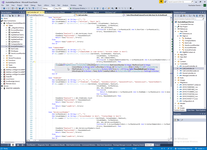Below is example of callback function.Result of using this function looking fine and satisfy me.And next my idea is create by Code Generation and T4 Text Templates calling each XLS loader because loader from loader is differ only by one literal (type T), column titles and need to expand some properties by Reflection in filter. But this is next story.

<00>  <01>  <02>  <03>  <04>  <05>  <06>  <07>  <08>  <09>  <10>  <11>  <12>  <13>  <14>  <15>  <16>  <17>  <18>  <19